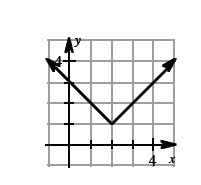### Home > CALC > Chapter 3 > Lesson 3.3.4 > Problem3-137

3-137.

For the absolute value function shown below, something interesting happens to the tangent of the function at $x = 2$. Can you draw more than one tangent at $x = 2$? Do you think this function has any valid tangents at $x = 2$?Consider the slope as $x→2^−$ and then consider the slope as $x→2^+$.

From the left, the slope appears to be $−1$.

From the right, what does the slope appear to be?